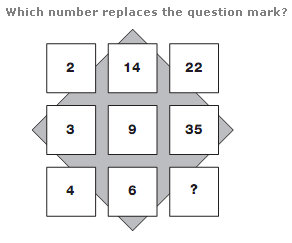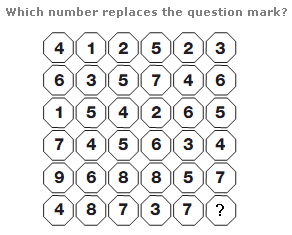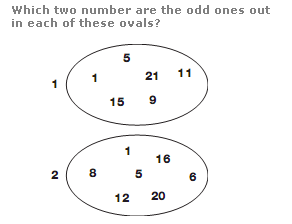# Puzzles - Number puzzles

Exercise : Number puzzles
97.:
56
Explanation
:
Starting at the top left and moving down, then to the right and up, in a snakes and ladders pattern, add the first two numbers together and subtract 1 to get the next in the sequence.

98.:
6
Explanation
:
Divide the whole diagram into 4 equal quarters, each comprising of a 3x3 square. The sequence of numbers in the top left quarter is repeated in each of the other quarters, but increasing by 1 each time as you move clockwise.

99.